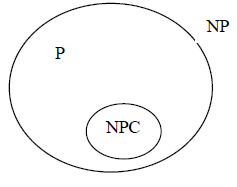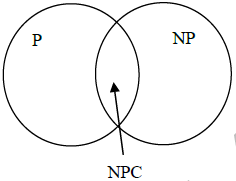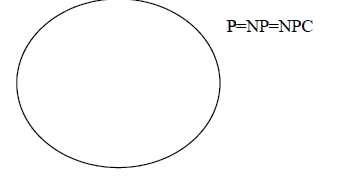Suppose a polynomial time algorithm is discovered that correctly computes the largest clique in a
given graph. In this scenario, which one of the following represents the correct Venn diagram of the
complexity classes P, NP and NP Complete (NPC)?
• a)• b)• c)• d)### Related Test## Can you answer this doubt?

People are searching for an answer to this question.